Atoms and Molecules - NCERT Questions
Q 1.

In a reaction, 5.3 g of sodium carbonate reacted with 6 g of ethanoic acid. The products were 2.2 g of carbon dioxide, 0.9 g of water and 8.2 g of sodium ethanoate. Show that these observations are in agreement with the law of conservation of mass.
Sodium carbonate + ethanoic acid sodium
ethanoate + carbon dioxide + water

SOLUTION:

Total mass of reactants = mass of sodium
carbonate + mass of ethanoic acid
= 5.3 g + 6 g = 11.3 g
Total mass of products = mass of sodium ethanoate + mass of carbon dioxide + mass
of water
= 8.2 g + 2.2 g + 0.9 g = 11.3 g
Thus, the mass of reactants is equal to the mass of products, therefore the observations are in agreement with the law of conservation of mass.

Q 2.

Hydrogen and oxygen combine in the ratio of 1 : 8 by mass to form water. What mass of oxygen gas would be required to react completely with 3 g of hydrogen gas?

SOLUTION:

1 g of hydrogen reacts with = 8 g of oxygen
∴ 3 g of hydrogen reacts with = 8 × 3 = 24 g of oxygen
Thus, 24 g of oxygen gas would be required to react completely with 3 g of hydrogen gas.

Q 3.

Which postulate of Dalton’s atomic theory is the result of the law of conservation of mass?

SOLUTION:

The postulate that “atoms can neither be created nor destroyed in a chemical reaction” is the result of the law of conservation of mass.

Q 4.

Which postulate of Dalton’s atomic theory can explain the law of definite proportions?

SOLUTION:

The postulate that “A chemical compound always consists of the same elements combined together in the same proportion by mass” is the law of definite proportions.

Q 5.

Define the atomic mass unit.

SOLUTION:

Atomic mass unit is defined as the mass unit equal to exactly one-twelfth (1/12th) of the mass of one atom of carbon-12. It is denoted by u (unified mass).
i.e. 1 u = 1.66 × 10–24 g

Q 6.

Why is it not possible to see an atom with naked eyes?

SOLUTION:

It is not possible to see an atom with naked eye because of its extremely small size (atomic radius is of the order of 10–10 m).

Q 7.

Write down the formulae of
(I) sodium oxide,
(ii) aluminium chloride,
(iii) sodium sulphide,
(iv) magnesium hydroxide

SOLUTION:

(I) Sodium oxide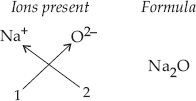(ii) Aluminium chloride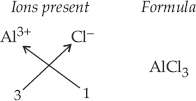(iii) Sodium sulphide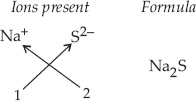(iv) Magnesium hydroxide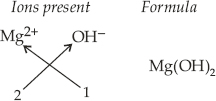Q 8.

Write down the names of compounds represented by the following formulae :
(I) Al2(SO4)3
(ii) CaCl2
(iii) K2SO4
(iv) KNO3
(v) CaCO3

SOLUTION:

(I) Aluminium sulphate
(ii) Calcium chloride
(iii) Potassium sulphate
(iv) Potassium nitrate
(v) Calcium carbonate

Q 9.

What is meant by the term chemical formulae?

SOLUTION:

The chemical formula of a compound is a symbolic representation of its composition. e.g., formula of calcium oxide.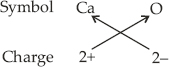Thus, chemical formula of calcium oxide is CaO.

Q 10.

How many atoms are present in a
(I) H2S molecule and
(ii) PO43– ion?

SOLUTION:

(I) 3 atoms because H2S molecule has two atoms of hydrogen and one atom of sulphur.
(ii) 5 atoms because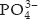ion has one atom of phosphorus and four atoms of oxygen.

Q 11.

Calculate the molecular masses of H2, O2, Cl2, CO2, CH4, C2H6, C2H4, NH3, CH3OH

SOLUTION:

Molecular mass of H2 = 2 × atomic mass of H
= 2 × 1 u = 2 u
Molecular mass of O2 = 2 × atomic mass of O
= 2 × 16 u = 32 u
Molecular mass of Cl2 = 2 × atomic mass of Cl
= 2 × 35.5 u = 71 u
Molecular mass of CO2 = atomic mass of C
+ 2 × atomic mass of O
= 12 + (2 × 16) = (12 + 32) u = 44 u
Molecular mass of CH4 = atomic mass of C
+ 4 × atomic mass of H
= 12 + (4 × 1) u = (12 + 4) u = 16 u
Molecular mass of C2H6 = 2 × atomic mass
of C + 6 × atomic mass of H
= (2 × 12 + 6 × 1) u = (24 + 6) u = 30 u
Molecular mass of C2H4 = 2 × atomic mass
of C + 4 × atomic mass of H
= (2 × 12 + 4 × 1) u = (24 + 4) u = 28 u
Molecular mass of NH3 = atomic mass of N
+ 3 × atomic mass of H
= (14 + 3 × 1) u = (14 + 3) u = 17 u
Molecular mass of CH3OH = atomic mass of C
+ 3 × atomic mass of H + atomic mass
of O + atomic mass of H
= (12 + 3 × 1 + 16 + 1) u = (12 + 3 + 17) u = 32 u

Q 12.

Calculate the formula unit masses of ZnO, Na2O, K2CO3. Given atomic masses of Zn = 65 u, Na = 23 u, K = 39 u, C = 12 u and O = 16 u

SOLUTION:

Formula unit mass of ZnO = atomic mass of Zn
+ atomic mass of O
= (65 + 16) u = 81 u
Formula unit mass of Na2O = 2 × atomic mass
of Na + atomic mass of O
= (2 × 23 + 16) u = 62 u
Formula unit mass of K2CO3
= 2 × atomic mass of K + atomic mass of C
+ 3 × atomic mass of O
= (2 × 39 + 12 + 3 × 16) u = (78 + 12 + 48) u
= 138 u

Q 13.

If one mole of carbon atoms weighs
12 gram, what is the mass (in gram) of
1 atom of carbon?

SOLUTION:

Molecular mass of carbon = 12 g
∵ 6.022 × 1023 atoms of carbon have mass
= 12 g
∴ 1 atom of carbon has mass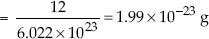Q 14.

Which has more number of atoms, 100 grams of sodium or 100 grams of iron (given atomic mass of Na = 23 u, Fe = 56 u)?

SOLUTION:

100 g of sodium :
Number of sodium atoms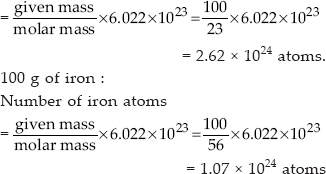Thus, 100 g of sodium has more atoms.

Q 15.

A 0.24 g sample of compound of oxygen and boron was found by analysis to contain 0.096 g of boron and 0.144 g of oxygen. Calculate the percentage composition of the compound by weight

SOLUTION:

We know that, % of any element in a compound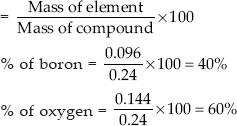Q 16.

When 3.0 g of carbon is burnt in 8.00 g oxygen, 11.00 g of carbon dioxide is produced. What mass of carbon dioxide will be formed when 3.00 g of carbon is burnt in 50.00 g of oxygen? Which law of chemical combination will govern your answer?

SOLUTION: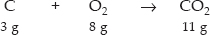Total mass of reactants = mass of C + mass
of O2 = 3 + 8 = 11 g
Total mass of reactants = total mass of
products
Hence, the law of conservation of mass is proved.
Further, it also shows carbon dioxide contains carbon and oxygen in a fixed ratio by mass, which is 3 : 8. Thus, it also proves the law of constant proportions. 3 g of carbon must also combine with 8 g of oxygen only. This means that (50 – 8) = 42 g of oxygen will remain unreacted.

Q 17.

What are polyatomic ions? Give examples

SOLUTION:

A polyatomic ion is a group of atoms carrying positive or negative charge.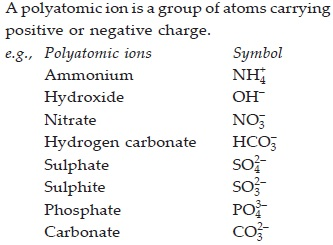Q 18.

Write the chemical formulae of the following :
(A) Magnesium chloride
(B) Calcium oxide
(C) Copper nitrate
(D) Aluminium chloride
(E) Calcium carbonate

SOLUTION:

(A) Magnesium chloride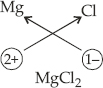(B) Calcium oxide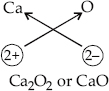(C) Copper nitrate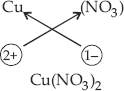(D) Aluminium chloride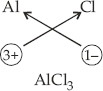(E) Calcium carbonate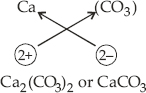Q 19.

Give the names of the elements present in the following compounds.
(A) Quick lime
(B) Hydrogen bromide
(C) Baking powder
(D) Potassium sulphate

SOLUTION:

(A) Quick lime is CaO. Elements present are calcium and oxygen.
(B) Hydrogen bromide is HBr. Elements present are hydrogen and bromine.
(C) Baking powder is NaHCO3. Elements present are sodium, hydrogen, carbon and oxygen.
(D) Potassium sulphate is K2SO4. Elements present are potassium, sulphur and oxygen.

Q 20.

Calculate the molar mass of the following substances.
(A) Ethyne, C2H2
(B) Sulphur molecule, S8
(C) Phosphorus molecule, P4 (atomic mass of phosphorus = 31)
(D) Hydrochloric acid, HCl
(E) Nitric acid, HNO3.

SOLUTION:

(A) Molar mass of C2H2 = 2 × atomic mass of C + 2 × atomic mass of H = (2 × 12 + 2 × 1) u = (24 + 2) u = 26 u
(B) Molar mass of S8 = 8 × atomic mass of S = (8 × 32) u = 256 u
(C) Molar mass of P4 = 4 × atomic mass of P = (4 × 31) u = 124 u
(D) Molar mass of HCl = atomic mass of H + atomic mass of Cl = (1 + 35.5) u = 36.5 u
(E) Molar mass of HNO3 = atomic mass of H+ atomic mass of N + 3 × atomic mass of O = (1 + 14 + 3 × 16) u = (1 + 14 + 48) u = 63 u

Q 21.

What is the mass of
(A) 1 mole of nitrogen atoms?
(B) 4 moles of aluminium atoms (atomic mass of aluminium = 27)?
(C) 10 moles of sodium sulphite (Na2SO3)?

SOLUTION:

(A) Mass of nitrogen atom
= number of moles × atomic mass
= 1 × 14 = 14 g
(B) Mass of aluminium atom
= 4 × atomic mass = 4 × 27 = 108 g
(C) Mass of Na2SO3 = 2 × 23 + 32 + 16 × 3
= 46 + 32 + 48 = 126 g
1 mole of Na2SO3 = 126 g
∴ 10 moles of Na2SO3 = (10 × 126) g
= 1260 g

Q 22.

Convert into mole.
(A) 12 g of oxygen gas
(B) 20 g of water
(C) 22 g of carbon dioxide

SOLUTION:

(A) Molar mass of O2 = 2 × 16 = 32 g
∴ Number of moles in 12 g of O2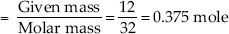(B) Molar mass of H2O = 2 × 1 + 16 = 18 g
∴ Number of moles in 20 g of H2O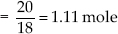(C) Molar mass of CO2 = 12 + 16 × 2
= 12 + 32 = 44 g
∴ Number of moles in 22 g of CO2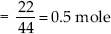Q 23.

What is the mass of
(A) 0.2 mole of oxygen atoms?
(B) 0.5 mole of water molecules?

SOLUTION:

(A) Mass of 1 mole of oxygen atom = 16 u
Mass of 0.2 mole of oxygen atoms
= (16 × 0.2) u = 3.2 u
(B) Mass of 1 mole of H2O molecule
= (1 × 2 + 16) = 18 u
Mass of 0.5 mole of H2O molecule
= (18 × 0.5) u = 9 u

Q 24.

Calculate the number of molecules of sulphur (S8) present in 16 g of solid sulphur?

SOLUTION:

Mass of 1 mole of S8 = 8 × 32 = 256 g
∴ 256 g of S8 contains = 6.022 × 1023
molecules
∴ 16 g of S8 contains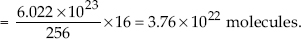Q 25.

Calculate the number of aluminium ions present in 0.051 g of aluminium oxide.
(Hint : The mass of an ion is the same as that of an atom of the same element. Atomic mass of Al = 27 u)

SOLUTION:

Molar mass of Al2O3 = 2 × 27 + 3 × 16
= 54 + 48 = 102 g
∴ 102 g of Al2O3 contains
= 2 × 6.022 × 1023 Al3+ ions
∴ 0.051 g of Al2O3 contains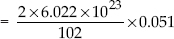= 6.022 × 1020 Al3+ ions.